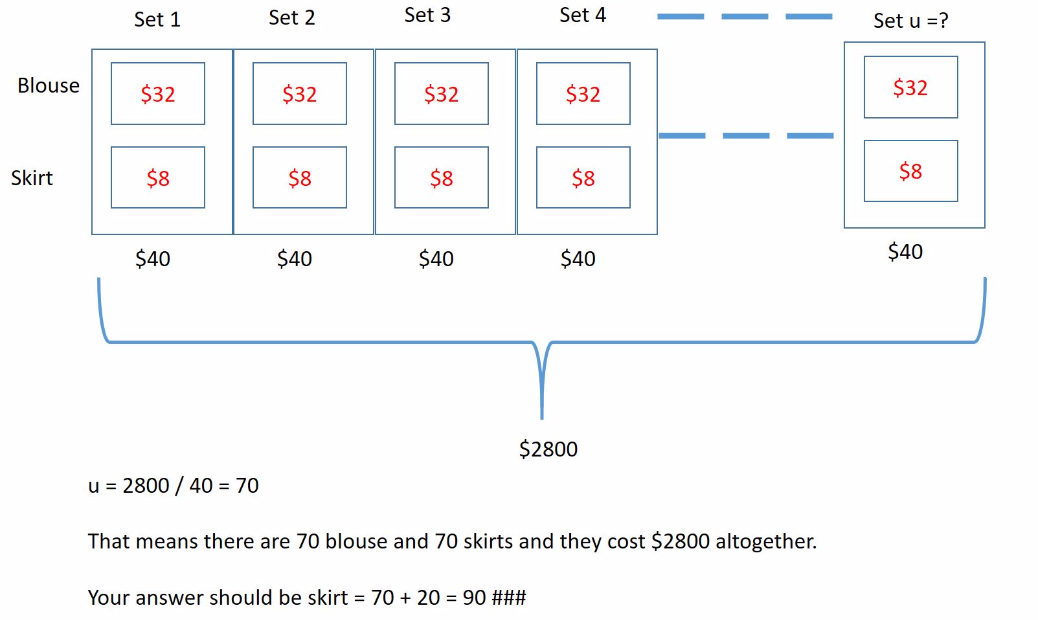# Question

Hi,

I get this question from the assessment book.

In a sale, Nancy spent \$2960 on some blouses and skirts. Each blouse cost \$32 and each skirt cost 25% as much as each blouse. She bought 20 more skirts than blouses. How many skirts did she buy?

Ans : 25% x \$32 = \$8

\$32 + \$8 + \$40

20 x \$8 = \$160

(\$2960 – \$160) ÷ 40 = 70

70 + 20 = 90

I cannot understand why the last part is divided by 40. Isn’t it blouses and skirts share the unit ?

My understanding is as follow:

(\$2960 – \$160)= \$2800

\$2800 ÷ 2 = \$1400

\$1400 + \$160 = \$1560

\$1560 ÷ 40 = 39

39 + 20 = 59

Hi Musicstar. I believe your confusion Is the cost of each skirt. The skirt does not cost 125%. It costs 25% of blouse and so it is only \$8 each. \$40 is 1 set of 1 blouse and 1 shirt.

Taking away the cost of excess 20 skirts (\$160), the remainder \$2800 is 70 sets of 1 blouse and 1 shirt (\$40 per set)

So there are 90 skirts (70+20).

Oh. Maybe your confusion is not that, could be you think the balance cost \$2800 is shared equally between equal no. of blouses and skirts.

If so this is a misconception. Think about those coins type questions.

5pcs of \$1-coins and 5pcs of 20c-coins in the piggy bank (total \$6) doesn’t mean \$3 of \$1-coins and \$3 of 20c-coins.

solution should be: total value/value per set = no. of sets

misconception: half total value / value per pc = no. of pcs

If your algebra is good, you can deduce the same thing from Khong’s workings / Ender’s picture.

2 Replies 1 Like

pleasant post, stay aware of this fascinating work. It truly regards realize that this subject is being secured likewise on this site so cheers for setting aside time to talk about this! Gym Near Me

0 Replies 0 LikesMy understanding is as follow:

(\$2960 – \$160)= \$2800

From here onwwards is wrong.

\$2800 ÷ 2 = \$1400          X
\$1400 + \$160 = \$1560    X
\$1560 ÷ 40 = 39                X
39 + 20 = 59                       X

When you minus \$160 and left \$2800, it does not mean the cost of the skirt is half of it. It just means for the same number of skirts and blouses, they cost \$2800 together.

Refer to my picture for understanding how the \$2800 is breakdown. You will realized that the skirt do not cost half of \$2800 as what you have initially thought.

0 Replies 2 Likes